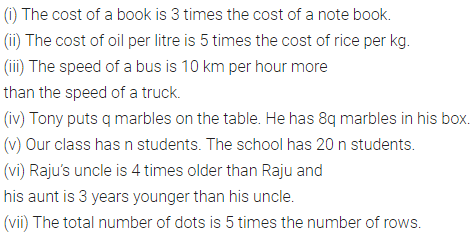# ML Aggarwal Class 6 Solutions for ICSE Maths Chapter 9 Algebra Ex 9.3

## ML Aggarwal Class 6 Solutions for ICSE Maths Chapter 9 Algebra Ex 9.3

Question 1.
Form four expressions with numbers 7, 5 and 8 (no variables) using operations of addition, subtraction or multiplication with the condition that every number should be used but not more than once.
Solution:Question 2.
Which out of the following are expressions with numbers only?
(i) 2y + 3
(ii) (7 × 20) – 82
(iii) 5 × (21 – 7) + 9 x 2
(iv) 5 -11 n
(v) (5 × 4) – 45 + p
(vi) 3 × (11 + 7) – 24 + 3
Solution: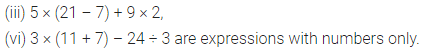Question 3.
Identify the operations (addition, subtraction, multiplication, division) informing the following expressions and tell how the expressions have been formed:
(i) x + 5
(ii) y – 7
(iii) 3z
(iv) $$\frac{p}{5}$$
(v) 2x + 17
(vi) 3y – 5
(vii) $$-7 m+\frac{2}{3}$$
(viii) $$\frac{x}{3}-15$$
Solution: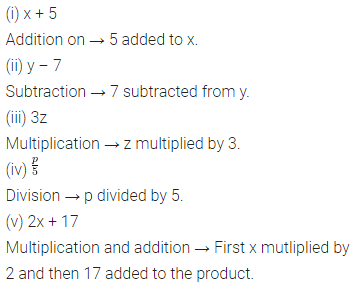Question 4.
Write expression for the following:
(ii) p subtracted from 7
(iii) p multiplied by 7
(iv) p divided by 7
(v) 7 divided by p
(vi) 7 subtracted from -m
(vii) p multiplied by -5
(viii) -p divided by 5
Solution: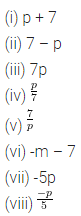Question 5.
Write an expression for the following:
(i) 11 added to 2 m
(ii) 11 subtracted from 2 m
(iii) 3 added to 5 times y
(iv) 3 subtracted from 5 times y
(v) y is multiplied by -8 and then 5 is added to the result
(vi) y is multiplied by 5 and then the result is subtracted from 16.
Solution: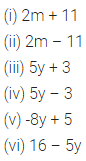Question 6.
Write the following in mathematical form using signs and symbols:
(i) 6 more than thrice a number x.
(ii) 7 taken away from y.
(iii) 3 less than quotient of x by y.
Solution: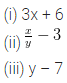Question 7.
Form six expressions using t and 4. Use not more than one number operation and every expression must have t in it.
Solution: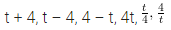Question 8.
Form expressions using y, 2 and 7. Use only two different number operations and every expression must have y in it.
Solution:Question 9.
A student scored x marks in English but the teacher deducted 5 marks for bad handwriting. What was the student’s final score in English?
Solution:Question 10.
Raju’s father’s age is 2 years more than 3 times Raju’s age. If Raju’s present age is y years, then what is his father’s age?
Solution: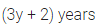Question 11.
Mohini is x years old. Express the following in algebraic form:
(i) three times Mohini’s age next year.
(ii) four times Mohini’s age 3 years ago.
(iii) the present age of Mohini’s uncle, if his uncle is 5 times as old as Mohini will be two years from now.
(iv) the present age of Mohini’s cousin, if her cousin is two years less than one-third of Mohini’s age five years ago.
Solution: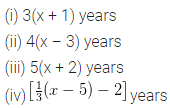Question 12.
A cuboidal box has height h cm. Its length is 5 times the height and breadth is 10 cm less than the length. Express the length and the breadth of the box in terms of the height.
Solution: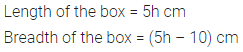Question 13.
A bus travels at v km per hour. It is going from Delhi to Jaipur. After the bus has travelled 5 hours, Jaipur is still 20 km away. What is the distance from Delhi to Jaipur?
Solution: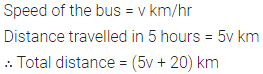Question 14.
Change the following statements using expressions into statements in ordinary language:
(i) A notebook cost ₹ p. A book costs ₹ 3p.
(ii) The cost of rice per kg is ₹ p. The cost of oil per litre is ₹5p.
(iii) The speed of a truck is v km per hour. The speed of a bus is (v + 10) km per hour.
(iv) Tony’s box contains 8 times the marbles he puts on the table.
(v) The total number of students in the school is 20 times that of our class.
(vi) Raju is x years old. His uncle is 4x years old and his aunt is (4x – 3) years old.
(vii) In arrangement of dots there are r rows. Each row contains 5 dots.
Solution: